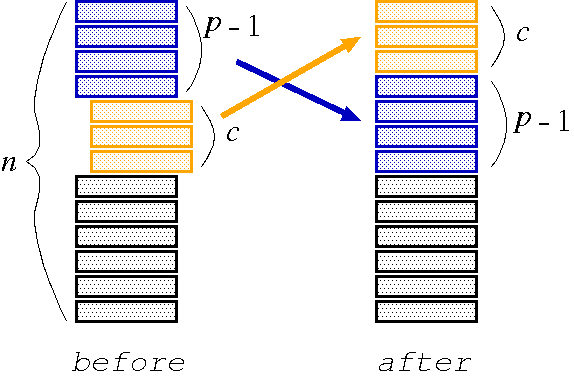Hanafuda Shuffle

Time Limit : 1 sec, Memory Limit : 131072 KB
Japanese version is here

Problem A: Hanafuda Shuffle

There are a number of ways to shuffle a deck of cards. Hanafuda shuffling for Japanese card game 'Hanafuda' is one such example. The following is how to perform Hanafuda shuffling.

There is a deck of n cards. Starting from the p-th card from the top of the deck, c cards are pulled out and put on the top of the deck, as shown in Figure 1. This operation, called a cutting operation, is repeated.

Write a program that simulates Hanafuda shuffling and answers which card will be finally placed on the top of the deck.Figure 1: Cutting operation

Input

The input consists of multiple data sets. Each data set starts with a line containing two positive integers n (1 <= n <= 50) and r (1 <= r <= 50); n and r are the number of cards in the deck and the number of cutting operations, respectively.

There are r more lines in the data set, each of which represents a cutting operation. These cutting operations are performed in the listed order. Each line contains two positive integers p and c (p + c <= n + 1). Starting from the p-th card from the top of the deck, c cards should be pulled out and put on the top.

The end of the input is indicated by a line which contains two zeros.

Each input line contains exactly two integers separated by a space character. There are no other characters in the line.

Output

For each data set in the input, your program should write the number of the top card after the shuffle. Assume that at the beginning the cards are numbered from 1 to n, from the bottom to the top. Each number should be written in a separate line without any superfluous characters such as leading or following spaces.

```5 2
3 1
3 1
10 3
1 10
10 1
8 3
0 0
```

Output for the Sample Input

```4
4
```

Source: ACM International Collegiate Programming Contest , Japan Domestic Contest, Ehime, Japan, 2004
http://www.ehime-u.ac.jp/ICPC/